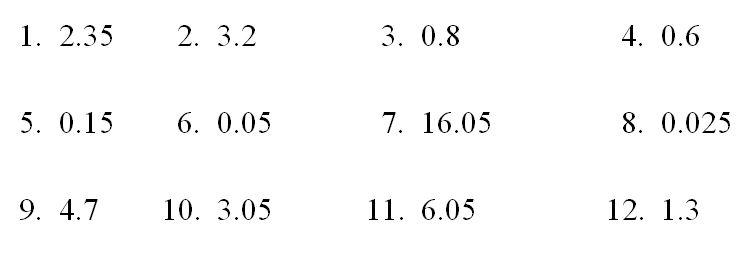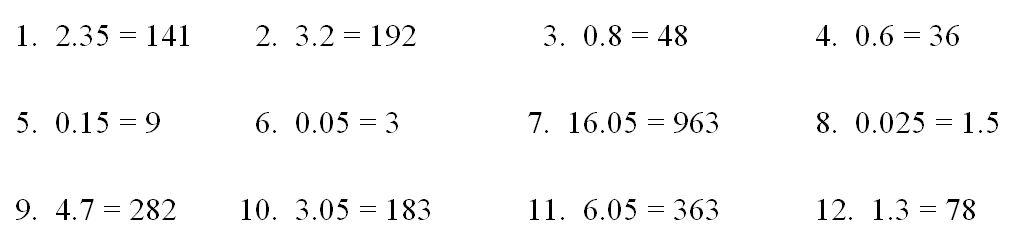# Chapter 2: Essential numeracy requirements for nursing

Cancel down 375/550 to its simplest form, dividing top and bottom until no further number is exactly divisible into both parts of the fraction.

15/22

Cancel down 625/890 to its simplest form, dividing top and bottom until no further number is exactly divisible into both parts of the fraction.

125/178

Cancel down 325/375 to its simplest form, dividing top and bottom until no further number is exactly divisible into both parts of the fraction.

13/15

Cancel down 64/96 to its simplest form, dividing top and bottom until no further number is exactly divisible into both parts of the fraction.

2/3

Cancel down 42/96 to its simplest form, dividing top and bottom until no further number is exactly divisible into both parts of the fraction.

7/16

Cancel down 24/69 to its simplest form, dividing top and bottom until no further number is exactly divisible into both parts of the fraction.

8/23

Cancel down 575/1275 to its simplest form, dividing top and bottom until no further number is exactly divisible into both parts of the fraction.

23/51

Cancel down 25/520 to its simplest form, dividing top and bottom until no further number is exactly divisible into both parts of the fraction.

5/104

Cancel down 45/475 to its simplest form, dividing top and bottom until no further number is exactly divisible into both parts of the fraction.

9/95

Cancel down 54/181 to its simplest form, dividing top and bottom until no further number is exactly divisible into both parts of the fraction.

54/181

Cancel down 21/24 to its simplest form, dividing top and bottom until no further number is exactly divisible into both parts of the fraction.

7/8

Cancel down 35/230 to its simplest form, dividing top and bottom until no further number is exactly divisible into both parts of the fraction.

7/46

Cancel down the following fractions to their simplest form, dividing top and bottom until no further number is exactly divisible into both parts of the fraction.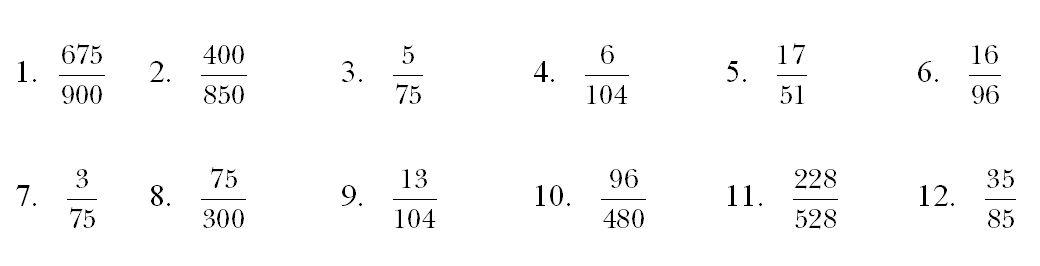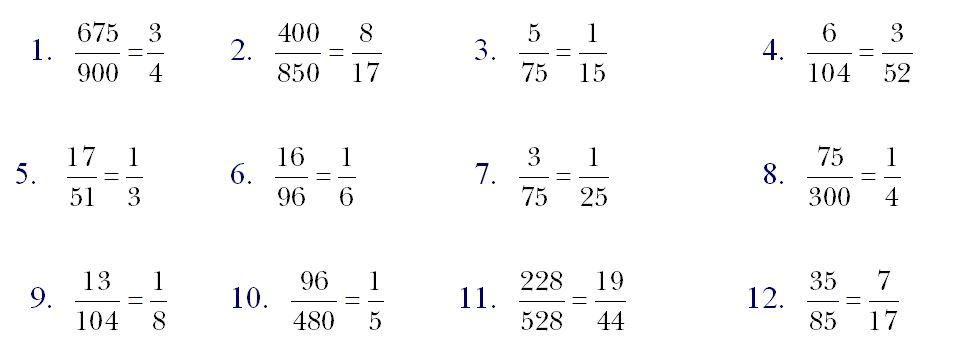Question type 2

Sebastian Contina needs to have 0.015g of medication per day. Calculate the weekly (7-day) requirement in grams.

0.105

Jess Crome needs to have 0.15g of medication per day. Calculate the weekly (7-day) requirement in grams.

1.05

Steven Gusty needs to have 0.0106g of medication per day. Calculate the weekly (7-day) requirement in grams.

0.0742

Helen Reckit needs to have 0.45g of medication per day. Calculate the weekly (7-day) requirement in grams.

3.15

Mark Strode needs to have 0.121g of medication per day. Calculate the weekly (7-day) requirement in grams.

0.847

Alison Barbara needs to have 0.05g of medication per day. Calculate the weekly (7-day) requirement in grams.

0.35

Falua McTaggart needs to have 0.25g of medication per day. Calculate the weekly (7-day) requirement in grams.

1.75

Arthur Dat needs to have 5.5g of medication per day. Calculate the weekly (7-day) requirement in grams.

38.5

Iain Burns needs to have 0.075g of medication per day. Calculate the weekly (7-day) requirement in grams.

0.525

As part of a stocktake of drugs at Arcadian Care and Respite Centre, you are to calculate the weekly (7-day) requirement in grams of the prescribed drug dosages for the following patients.

1.  Jonathon Sloe needs to have 0.035g per day

2.  Jennie Christian needs to have 0.255g per day

3.  Steven Grumbles needs to have 5.07g per day

Later on your placement you carry out a similar stocktake at Glad Tidings Rehabilitation Clinic. Again you need to calculate the weekly (7-day) requirement in grams of drugs for the following patients.

4.  Freddie Flumpit needs to have 1.45g per day

5.  Pauline Poorlie needs to have 0.028g per day

6.  Nate Burgen needs to have 3.45g per day

7.  Fay Dunnit needs to have 0.020g per day

8.  Billy Knotear needs to have 6.5g per day

9.  Iama Lookin needs to have 3.75g per day

1.   Jonathon Sloe needs to have 0.035g per day so for 7 days needs 0.245g

2.   Jennie Christian needs to have 0.255g per day so for 7 days needs 1.785g

3.   Steven Grumbles needs to have 5.07g per day so for 7 days needs 35.49g

4.   Freddie Flumpit needs to have 1.45g per day so for 7 days needs 10.15g

5.   Pauline Poorlie needs to have 0.028g per day so for 7 days needs 0.196g

6.   Nate Burgen needs to have 3.45g per day so for 7 days needs 24.15g

7.   Fay Dunnit needs to have 0.020g per day so for 7 days needs 0.14g

8.   Billy Knotear needs to have 6.5g per day so for 7 days needs 45.5g

9.   Iama Lookin needs to have 3.75g per day so for 7 days needs 26.25g

Question type 3

The label on a bottle of syrup mixture reads 9.6mcg/6ml. Calculate in mcg/ml the amount of drug weight in 1 millilitre of this syrup mixture.

1.6

The label on a bottle of syrup mixture reads 56.7mg/7ml. Calculate in mg/ml the amount of drug weight in 1 millilitre of this syrup mixture.

8.1

The label on a bottle of syrup mixture reads 97.5mcg/5ml. Calculate in mcg/ml the amount of drug weight in 1 millilitre of this syrup mixture.

19.5

The label on a bottle of syrup mixture reads 1.2g/8ml. Calculate in g/ml the amount of drug weight in 1 millilitre of this syrup mixture.

0.15

The label on a bottle of syrup mixture reads 1.5mg/4ml. Calculate in mg/ml the amount of drug weight in 1 millilitre of this syrup mixture.

0.375

The label on a bottle of syrup mixture reads 7.04mg/8ml. Calculate in mg/ml the amount of drug weight in 1 millilitre of this syrup mixture.

0.88

The label on a bottle of syrup mixture reads 9.2mcg/4ml. Calculate in mcg/ml the amount of drug weight in 1 millilitre of this syrup mixture.

2.3

The label on a bottle of syrup mixture reads 55.055g/55ml. Calculate in g/ml the amount of drug weight in 1 millilitre of this syrup mixture.

1.001

The label on a bottle of syrup mixture reads 0.072mg/3ml. Calculate in mg/ml the amount of drug weight in 1 millilitre of this syrup mixture.

0.024

Calculate the amount of drug weight in 1 millilitre of the following syrup mixtures.

1.  13.2mcg/6ml        2.   57.4mg/7ml            3. 16.5mcg/5ml

4.  2.4g/8ml               5.   2.04mg/4ml            6. 17.6mg/5ml

7.  1.72mcg/4ml        8.   3.25g/5ml               9. 0.072mg/12ml

1. 2.2mcg/ml              2. 8.2mg/ml          3. 3.3mcg/ml

4. 0.3g/ml                  5. 0.51mg/ml        6. 3.52mg/ml

7. 0.43mcg/ml           8. 0.65g/ml            9. 0.006mg/ml

Question type 4

Convert 0.50 into a fraction in its simplest or lowest form.

1/2

Convert 0.25 into a fraction in its simplest or lowest form.

1/4

Convert 0.375 into a fraction in its simplest or lowest form.

3/8

Convert 0.08 into a fraction in its simplest or lowest form.

2/25

Convert 0.044 into a fraction in its simplest or lowest form.

11/250

Convert 0.04 into a fraction in its simplest or lowest form.

1/25

Convert 0.125 into a fraction in its simplest or lowest form.

1/8

Convert 0.075 into a fraction in its simplest or lowest form.

3/40

Convert 0.010 into a fraction in its simplest or lowest form.

1/100

Convert 0.072 into a fraction in its simplest or lowest form.

9/125

Convert 0.200 into a fraction in its simplest or lowest form.

1/5

Convert 0.875 into a fraction in its simplest or lowest form.

7

Convert the following decimals to fractions in their simplest or lowest form.

1. 0.05          2. 0.075              3. 0.35               4. 0.025

5. 0.84          6. 0.375              7. 0.02                8. 0.045

9. 0.605       10. 0.875             11. 0.700             12. 0.65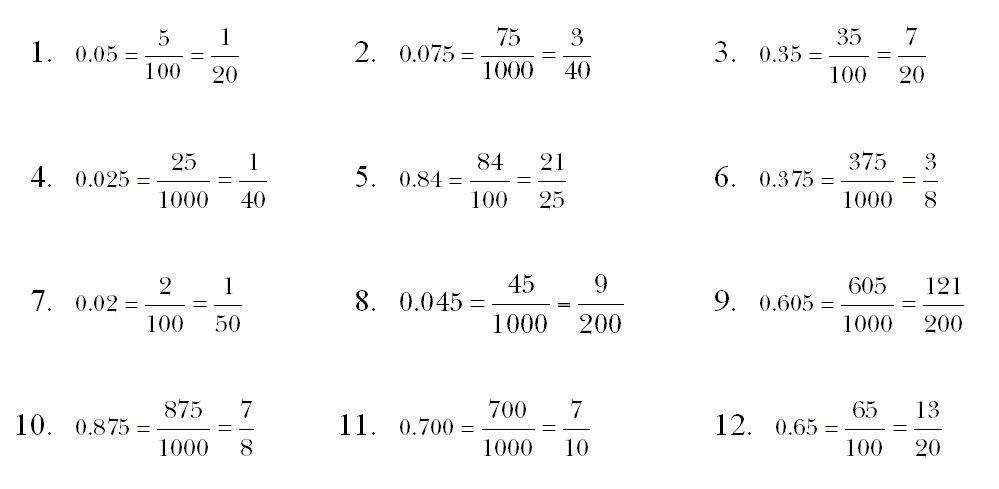Question type 5

What is 8.1 ÷ 0.9

9

What is 1.5 ÷ 0.05

30

What is 0.075 ÷ 0.0025

30

What is 10 ÷ 2.5

4

What is 0.325 ÷ 0.025

13

What is 0.090 ÷ 0.015

6

1.  27.9 ÷ 9.0          2.         7.5 ÷ 0.05          3.         0.0175 ÷ 0.05

4.  15 ÷ 2.5             5.         0.875 ÷ 0.25      6.         0.090 ÷ 1.6

1. 27.9 ÷ 9.0 = 3.1           2. 7.5 ÷ 0.05 = 150             3. 0.0175 ÷ 0.05 = 0.35

4. 15 ÷ 2.5 = 6                 5. 0.875 ÷ 0.25 = 3.5          6. 0.090 ÷ 1.6 = 0.05625

Question type 6

Convert the fraction 13/4 to a decimal answer.

3.25

Convert the fraction 10/8 to a decimal answer.

1.25

Convert the fraction 22/4 to a decimal answer.

5.5

Convert the fraction 7/4 to a decimal answer.

1.75

Convert the fraction 15/4 to a decimal answer.

3.75

Convert the fraction 26/4 to a decimal answer.

6.5

Convert the fraction 17/8 to a decimal answer.

2.125

Convert the fraction 34/8 to a decimal answer.

4.25

Convert the fraction 17/4 to a decimal answer.

4.25

Convert the fraction 12/5 to a decimal answer.

2.4

Convert the fraction 21/10 to a decimal answer.

2.1

Convert the fraction 27/5 to a decimal answer.

5.4

Convert these fractions to decimals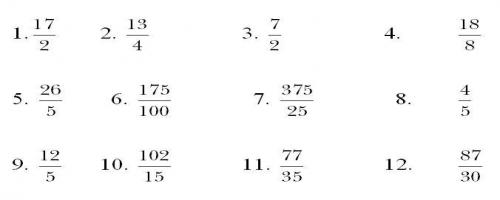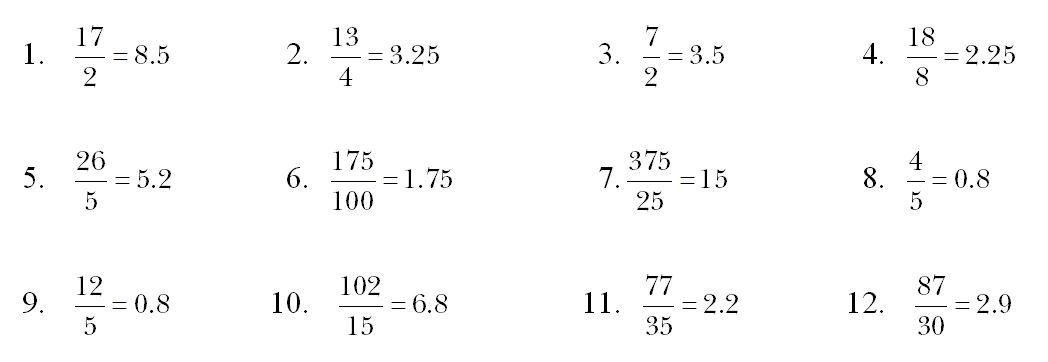Question type 7

Round 1.7 to the nearest whole number.

2

Round 11.92 to the nearest whole number.

12

Round 39.6 to the nearest whole number.

40

Round 60.4 to the nearest whole number.

60

Round 63.7 to the nearest whole number.

64

Round 17.18 to the nearest whole number.

17

Round 9.9 to the nearest whole number.

10

Round each of the following values to the nearest whole number.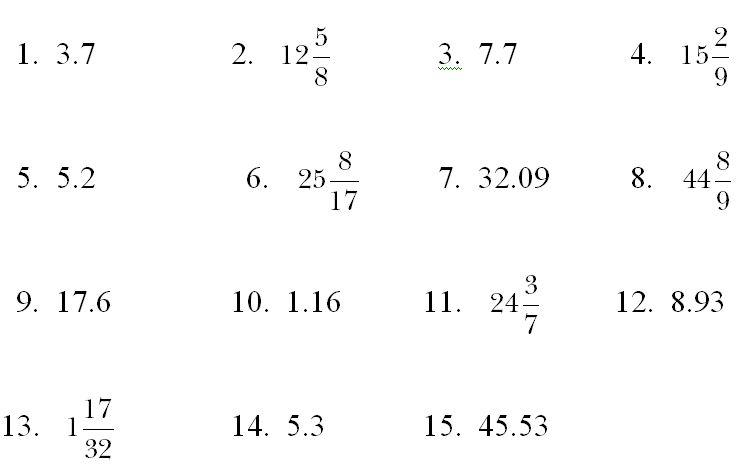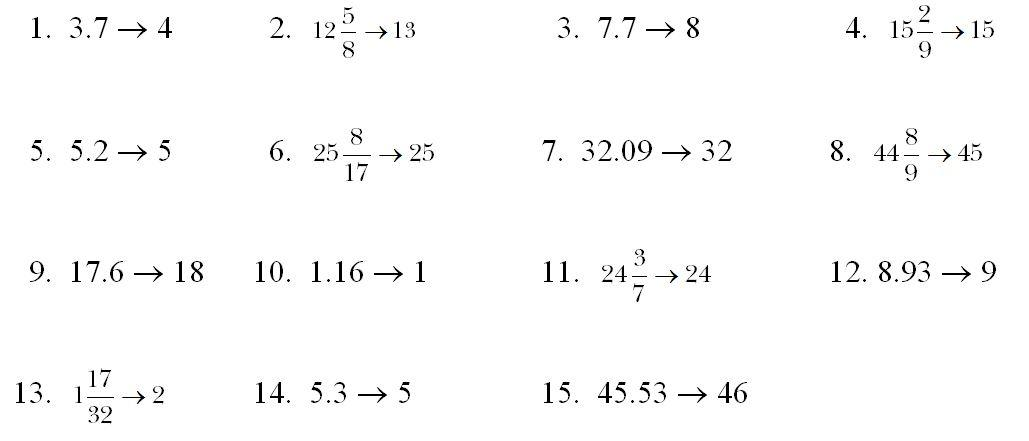Question type 8

Convert this fraction of hours into a number of minutes: 4/6

40

Convert this fraction of hours into a number of minutes: 3/2

90

Convert this fraction of hours into a number of minutes: 3/5

36

Convert this fraction of hours into a number of minutes: 4/15

16

Convert this fraction of hours into a number of minutes: 7/6

70

Convert this fraction of hours into a number of minutes: 4/10

24

Convert this fraction of hours into a number of minutes: 15/20

45

Convert this fraction of hours into a number of minutes: 4/5

48

Convert the following fractions of hours to a number of minutes.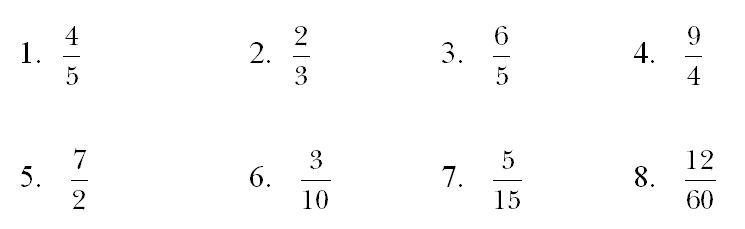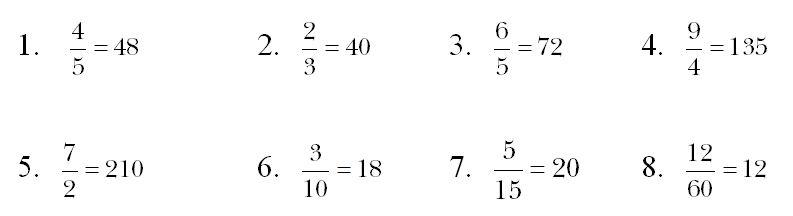Question type 9

Janet Barko is under observation and consumed the following: 275ml + 75ml + 250ml + 480ml. The following amounts were recorded as outputs: 305ml + 212ml + 375ml + 125ml. Find the final fluid balance in millilitres.

63

Eddese Grim is under observation and consumed the following: 365ml + 305ml + 250ml + 225ml. The following amounts were recorded as outputs: 375ml + 160ml + 185ml + 215ml. Find the final fluid balance in millilitres.

210

Ali Barker is under observation and consumed the following: 485ml + 350ml + 275ml + 176ml. The following amounts were recorded as outputs: 450ml + 365ml + 245ml + 175ml. Find the final fluid balance in millilitres

51

The following patients are all under observation. For each patient, find the total fluid intake, the total fluid output, and hence the fluid balance.

1.  Jackson Dunnit consumed the following:

235ml + 125ml + 175ml + 480ml

The following amounts were recorded as outputs:

275ml + 157ml + 345ml + 165ml

2.  Ed Wazzear consumed the following:

315ml + 380ml + 275ml + 220ml

The following amounts were recorded as outputs:

295ml + 165ml + 153ml + 225ml

3.  Ali Barba consumed the following:

485ml + 575ml + 175ml + 376ml

The following amounts were recorded as outputs:

450ml + 525ml + 315ml + 375ml

1.   Jackson Dunnit consumed the following:

235ml + 125ml + 175ml + 480ml

The following amounts were recorded as outputs:

275ml + 157ml + 345ml + 165ml

1015 - 942 = A positive balance of 73ml

2.   Ed Wazzear consumed the following:

315ml + 380ml + 275ml + 220ml

The following amounts were recorded as outputs:

295ml + 165ml + 153ml + 225ml

1190 - 838 = A positive balance of 352ml

3.   Ali Barba consumed the following:

485ml + 575ml + 175ml + 376ml

The following amounts were recorded as outputs:

450ml + 525ml + 315ml + 375ml

1611 - 1665 = A negative balance of 54ml

Question type 10

Covert the following hours, shown as a decimal, into a total number of minutes: 0.55

33

Covert the following hours, shown as a decimal, into a total number of minutes: 3.3

198

Covert the following hours, shown as a decimal, into a total number of minutes: 3.1.

186

Covert the following hours, shown as a decimal, into a total number of minutes: 0.25

15

Covert the following hours, shown as a decimal, into a total number of minutes: 12.2

732

Covert the following hours, shown as a decimal, into a total number of minutes: 5.45

327

Covert the following hours, shown as a decimal, into a total number of minutes: 5.8

348

Covert the following hours, shown as a decimal, into a total number of minutes: 0.3

18

Covert the following hours, shown as a decimal, into a total number of minutes: 11.5

690

Covert the following hours, shown as a decimal, into a total number of minutes: 4.6

276

Covert the following hours, shown as a decimal, into a total number of minutes: 7.2

432

Covert the following hours, shown as a decimal, into a total number of minutes: 0.75

45